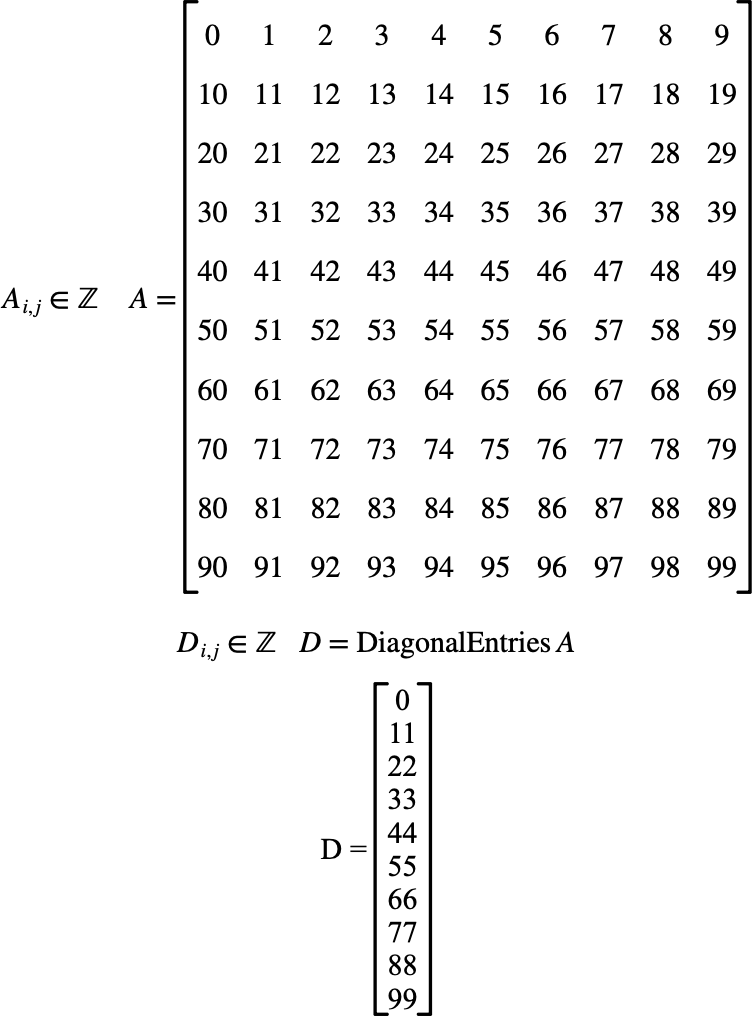# $$\text{DiagonalEntries}$$¶

You can use this function to obtain the diagnonal entries of a matrix.

You can use the \diagent backslash command to insert this function.

The following variants of this function are available:

• $$\text{ matrix } \text{DiagonalEntries} \left ( \text{<matrix>} \right )$$

The returned matrix will be the same type as $$\text{<matrix>}$$. The returned value will be a column matrix with one entry per diagnonal entry in the the matrix.

Figure 124 shows the basic use of the $$\text{DiagonalEntries}$$ function.Figure 124 Example Use of The DiagonalEntries Function